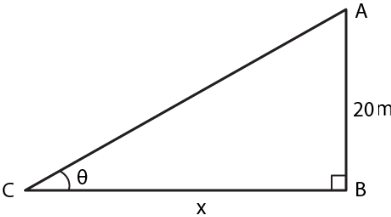Guru

# A vertical tower is 20 m high. A man standing at some distance from the tower knows that the cosine of the angle of elevation of the top of the tower is 0.53. How far is he standing from the foot of the tower?

• 0

sir this is the question from the book -ML aggarwal( avichal publication) class 10th , chapter20 , heights and distances
we have the inoformation about the question that A vertical tower is 20 m high.
A man standing at some distance from the tower knows that
the cosine of the angle of elevation of the top of the tower is 0.53.
How far is he standing from the foot of the tower?
an important question from the chapter
question no 11 , heights and distances , ICSE board, ML Aggarwal

Share

1. Consider AB as the tower

Take a man C stands at a distance x m from the foot of the tower

cos θ = 0.53We know that

Height of the tower AB = 20 m

cos θ = 0.53

So we get

θ = 580

Let us take

tan θ = AB/CB

Substituting the values

tan 580 = 20/x

So we get

1.6003 = 20/x

By cross multiplication

x = 20/1.6003

x = 12.49 = 12.5 m

Hence, the height of the tower is 12.5 m.

• 0